# NCERT Solutions for Class 6 Maths Chapter 13 Introduction to Symmetry

## NCERT Solutions for Class 6 Maths Chapter 13 Introduction to Symmetry

Today in our article you will be told that when your ncert solutions for class 6 maths chapter 13 Introduction to Symmetry will be released and you will be provided complete information about it. In our article, you will also be given complete information about the model paper. Also, you will be provided complete information about the model paper download process in our article. So please read it carefully till the end and stay connected with our website till the end.

ncert solutions for class 6 maths Chapter 13 Introduction to Symmetry

## Exercise 13.1

1. List any four symmetrical objects from your home or school.

Solutions:

The four symmetrical objects are the blackboard, the tabletop, a pair of scissors, the computer disc, etc.

2. For the given figure, which one is the mirror line, l1 or l2?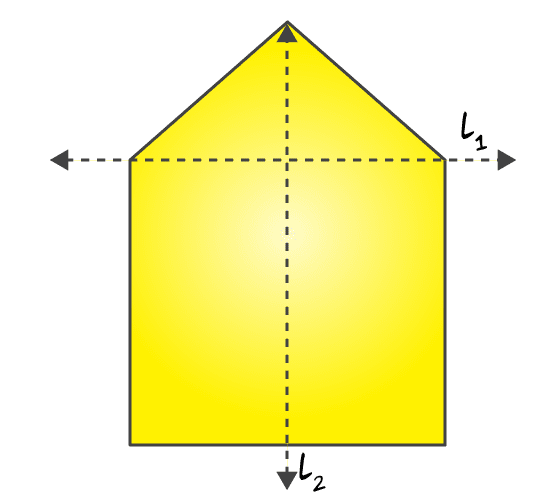Solutions:

l2 is the mirror line of the figure. When the given figure is folded about the line l2 the left part exactly cover the right part and vice versa

3. Identify the shapes given below. Check whether they are symmetric or not. Draw the line of symmetry as well.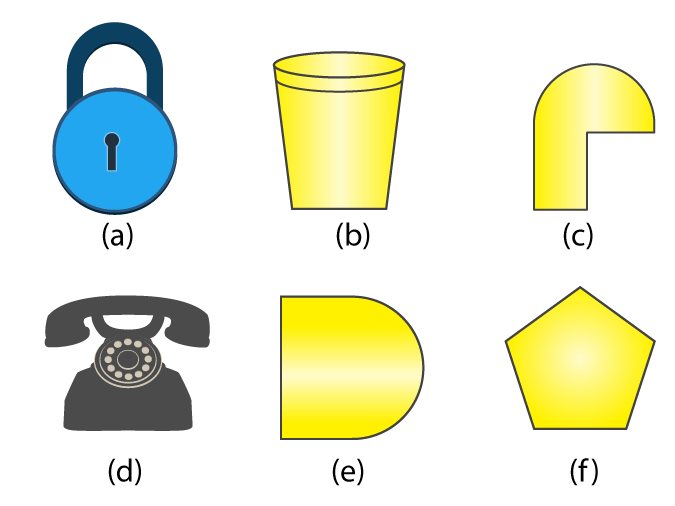Solutions:

(a) Yes. It is a symmetric

(b) Yes. It is a symmetric

(c) No, it is not a symmetric

(d) Yes. It is a symmetric

(e) Yes. It is a symmetric

(f) Yes. It is a symmetric

The following figures given below are the line of symmetry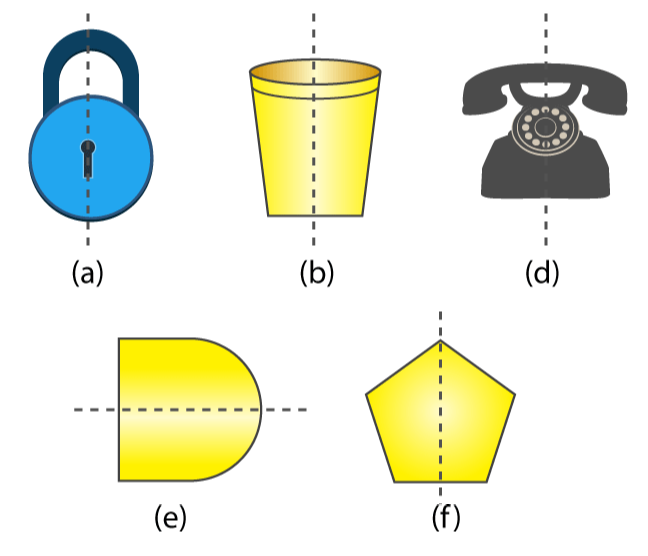4. Copy the following on a squared paper. A square paper is what you would have used in your arithmetic notebook in earlier classes. Then complete them such that the dotted line is the line of symmetry.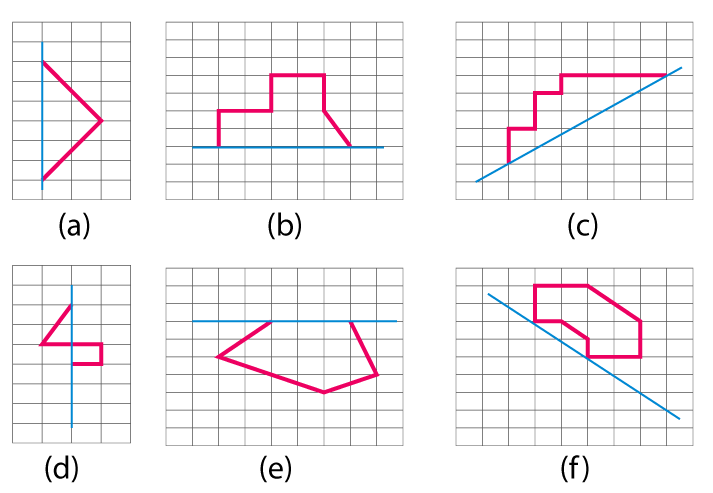Solutions:

To make the dotted line a line of symmetry the given figure may be drawn as follows

(a)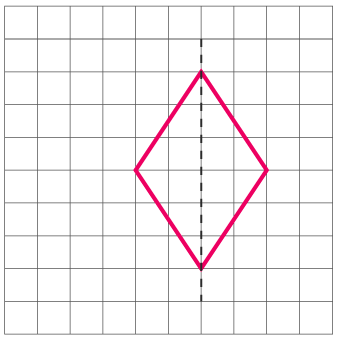(b)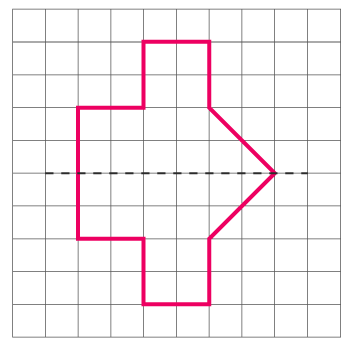(c)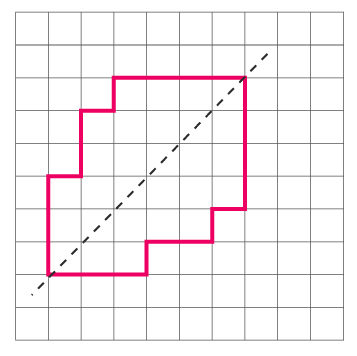(d)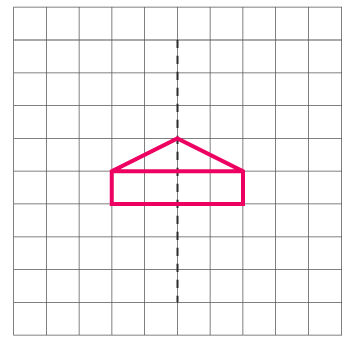(e)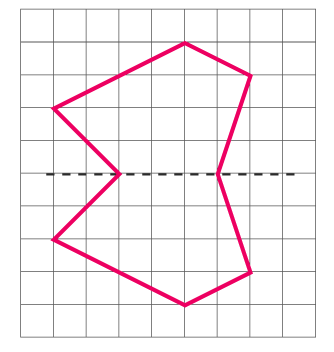(f)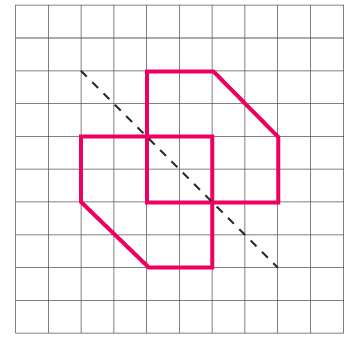5. In the figure, l is the line of symmetry. Complete the diagram to make it symmetric.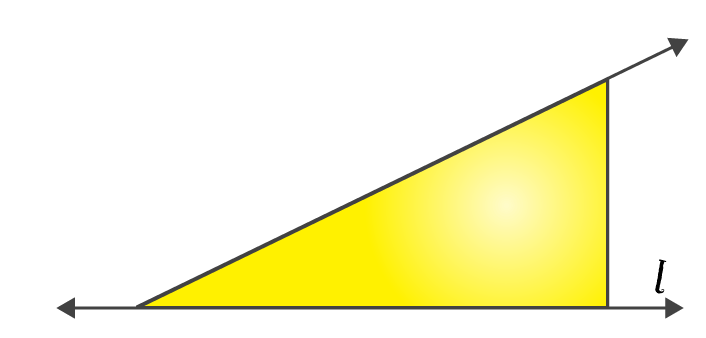Solutions:

The below figure is the complete diagram to make it symmetric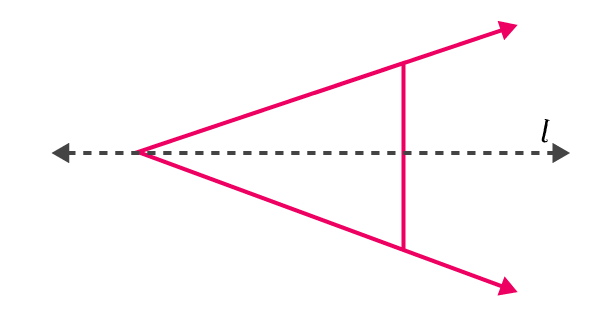6. In the figure, l is the line of symmetry. Draw the image of the triangle and complete the diagram so that it becomes symmetric.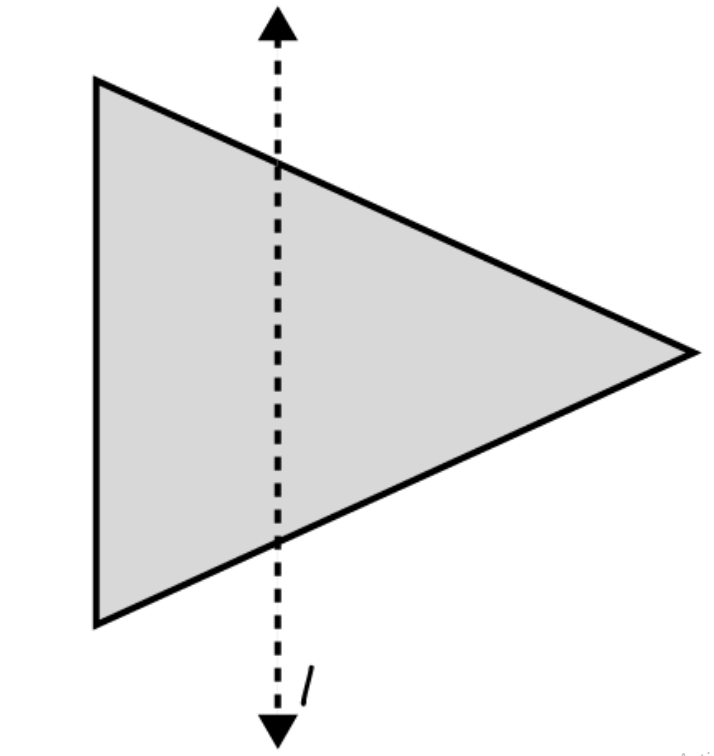Solutions:

The required triangle may be drawn as following to make it symmetric## Exercise 13.2

1. Find the number of lines of symmetry for each of the following shapes :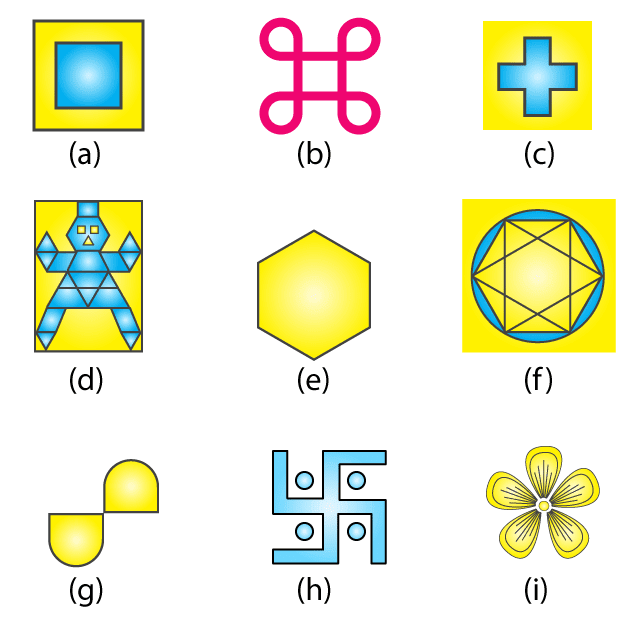Solutions:

(a) For the given figure there are 4 lines of symmetry.

(b) For the given figure there are 4 lines of symmetry.

(c) For the given figure there are 4 lines of symmetry.

(d) For the given figure there is only 1 line of symmetry.

(e) For the given figure there are 6 lines of symmetry.

(f) For the given figure there are 6 lines of symmetry.

(g) For the given figure there is no line of symmetry.

(h) For the given figure there is no line of symmetry.

(i) For the given figure there are 3 lines of symmetry.

Now we may observe the lines of symmetry in the above figures as follows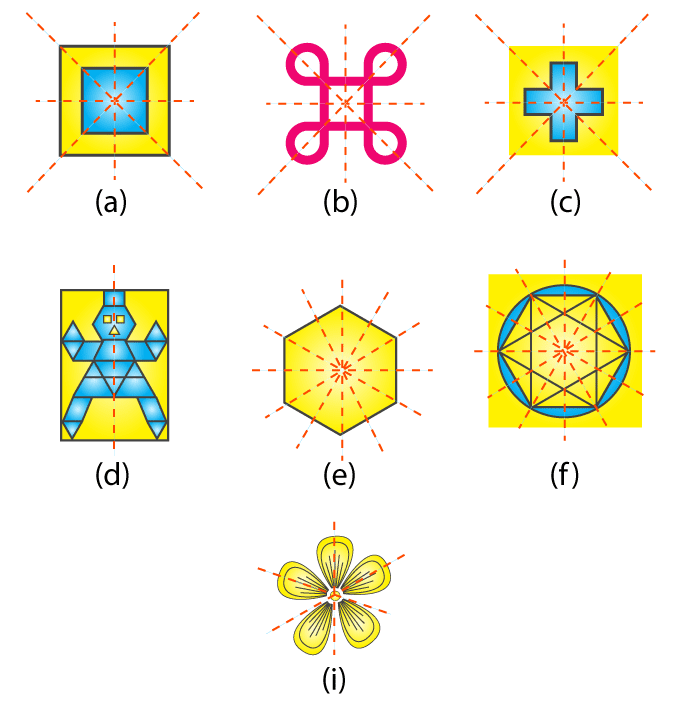2. Copy the triangle in each of the following figures on squared paper. In each case, draw the line(s) of symmetry, if any and identify the type of triangle. (Some of you may like to trace the figures and try paper folding first!)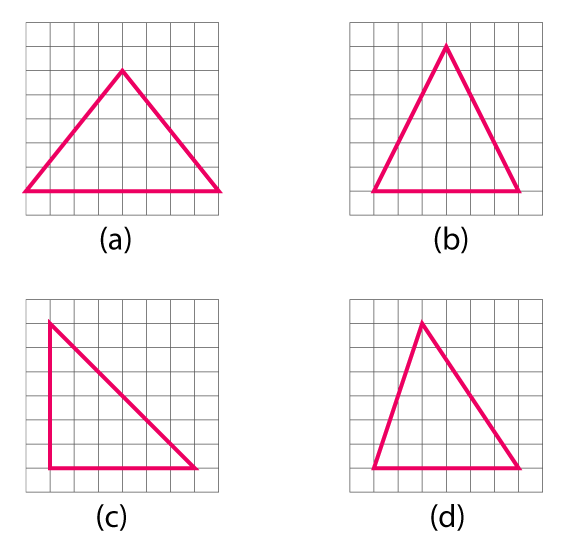Solutions:

(a) The given triangle is an isosceles triangle. Here it will be only 1 line of symmetry.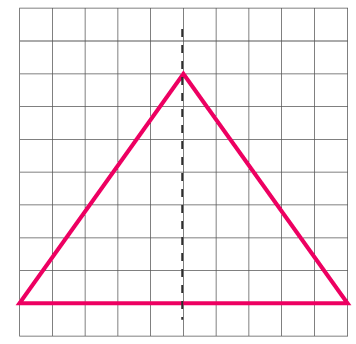(b) The given triangle is an isosceles triangle. Here it will be only 1 line of symmetry.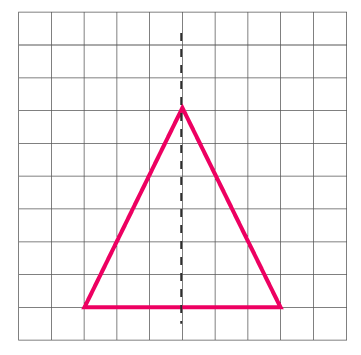(c) The given triangle is a right angled triangle. Here it will be only 1 line of symmetry.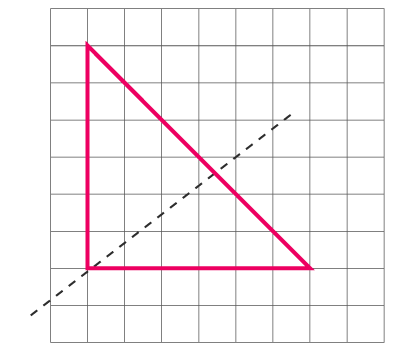(d) The given triangle is a scalene triangle. Here it will be no line of symmetry.

3. Complete the following table.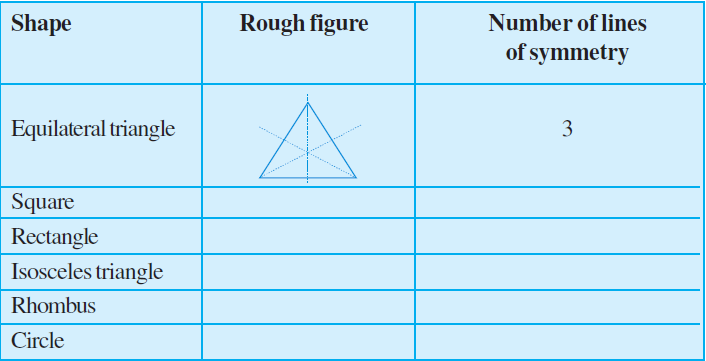Solutions:

The given table is completed as follows: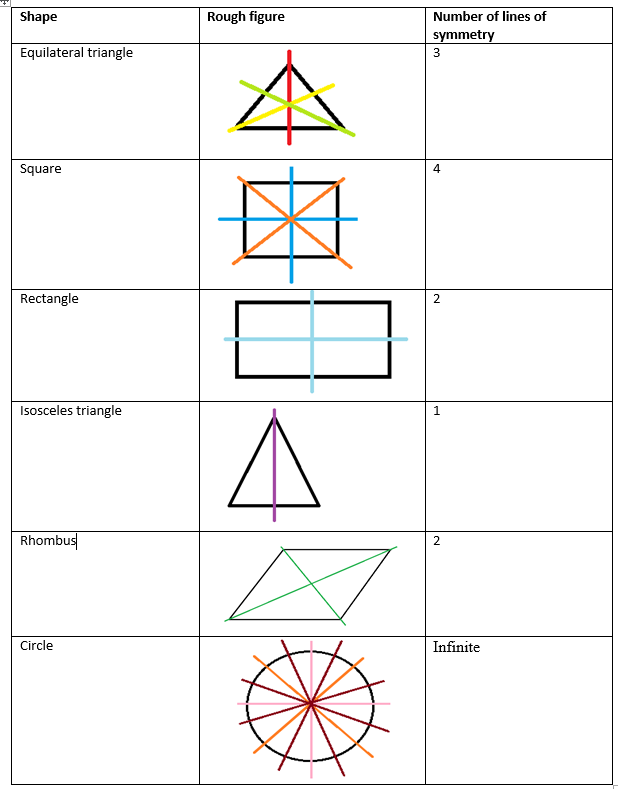In case of circles we find infinite lines. Here some lines of symmetry are drawn. Similarly, we can draw more symmetric lines for the circle.

4. Can you draw a triangle which has

(a) exactly one line of symmetry?

(b) exactly two lines of symmetry?

(c) exactly three lines of symmetry?

(d) no lines of symmetry?

Sketch a rough figure in each case.

Solutions:

(a) Yes, we can draw an isosceles triangle that has only 1 line of symmetry.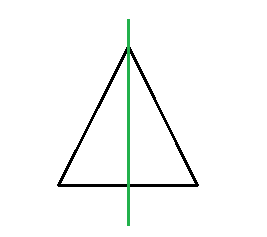(b) No, we cannot draw a triangle that has 2 lines of symmetry.

(c) Yes, we can draw an equilateral triangle that has 3 lines of symmetry.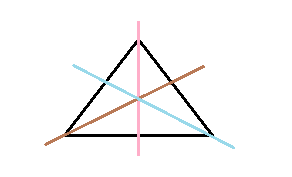(d) Yes, we can draw a scalene triangle which has no line of symmetry.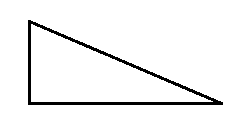5. On a squared paper, sketch the following:

(a) A triangle with a horizontal line of symmetry but no vertical line of symmetry.

(b) A quadrilateral with both horizontal and vertical lines of symmetry.

(c) A quadrilateral with a horizontal line of symmetry but no vertical line of symmetry.

(d) A hexagon with exactly two lines of symmetry.

(e) A hexagon with six lines of symmetry.

Solutions:

(a) A triangle with a horizontal line of symmetry but no vertical line of symmetry can be drawn as follows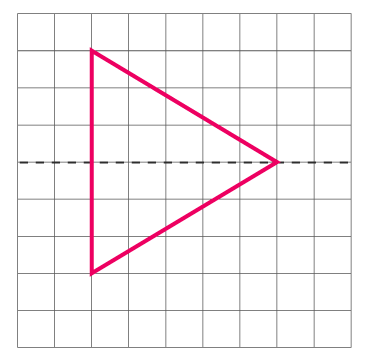(b) A quadrilateral with both horizontal and vertical lines of symmetry can be drawn as follows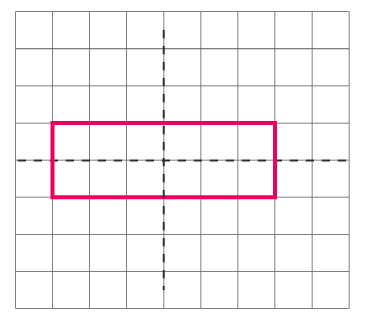(c) A quadrilateral with a horizontal line of symmetry but no vertical line of symmetry can be sketched as follows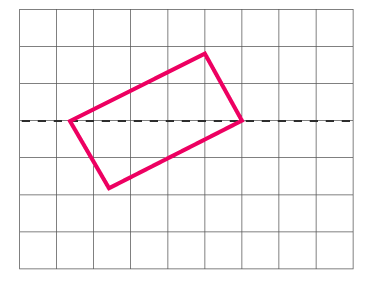(d) A hexagon with exactly two lines of symmetry may be drawn as follows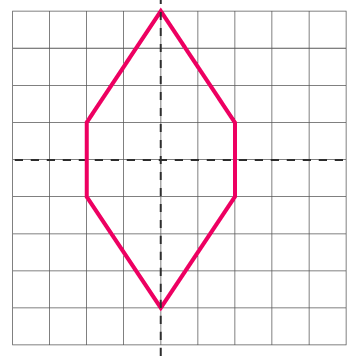(e) A hexagon with 6 lines of symmetry may be drawn as follows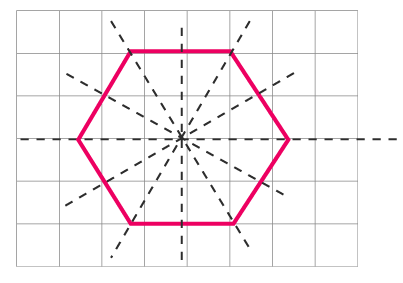6. Trace each figure and draw the lines of symmetry, if any: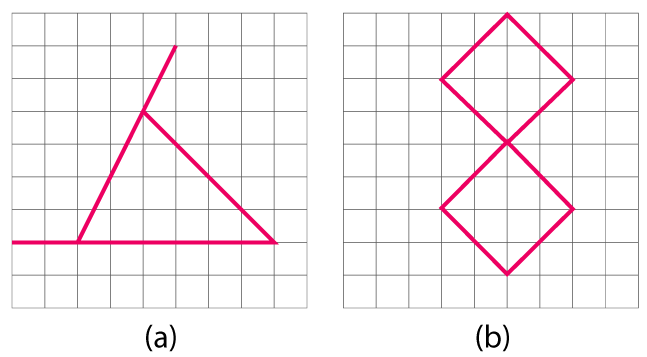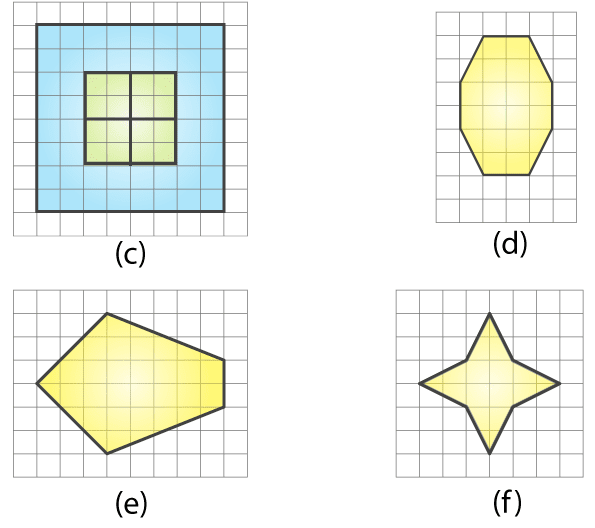Solutions:

(a) The given figure is an isosceles triangle. Hence, there will be 1 line of symmetry.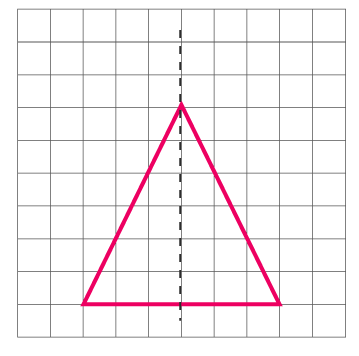(b) The given figure has two lines of symmetry.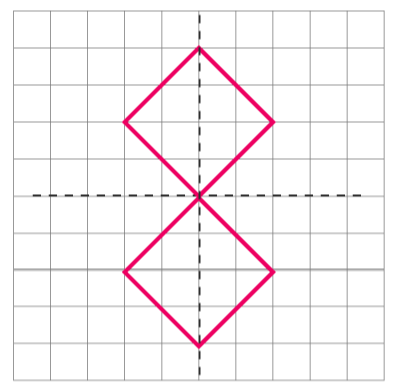(c) The given figure has 4 lines of symmetry.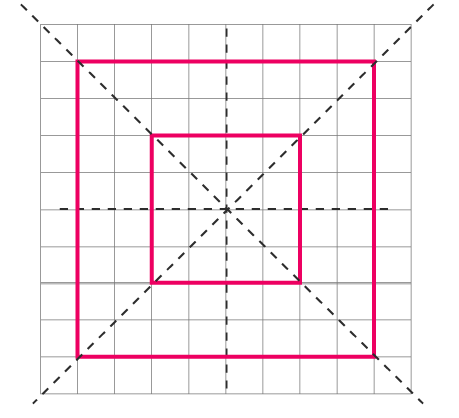(d) The given figure is an octagonal which has 2 lines of symmetry.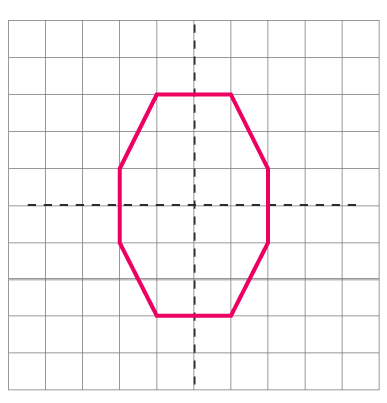(e) The given figure has only 1 line of symmetry.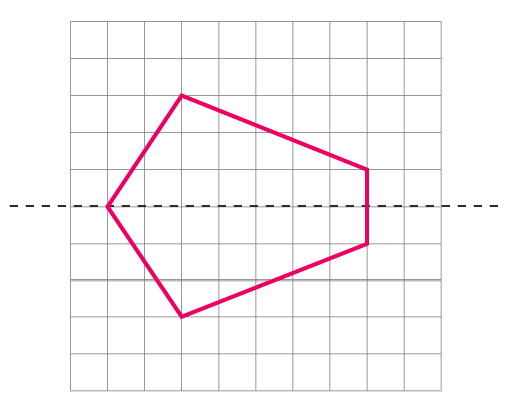(f) The given figure has 4 lines of symmetry.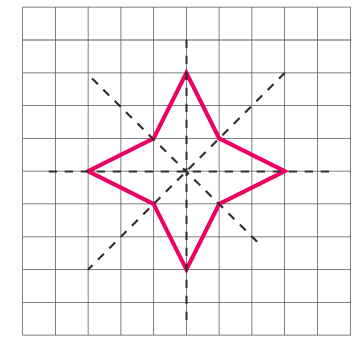7. Consider the letters of English alphabets, A to Z. List among them the letters which have

(a) vertical lines of symmetry (like A)

(b) horizontal lines of symmetry (like B)

(c) no lines of symmetry (like Q)

Solutions:

(a) Vertical lines of symmetry are A, H, I, M, O, T, U, V, W, X, Y.

(b) Horizontal lines of symmetry are B, C, D, E, H, I, K, O, X.

(c) No lines of symmetry are F, G, J, L, N, P, Q, R, S, Z.

8. Given here are figures of a few folded sheets and designs drawn about the fold. In each case, draw a rough diagram of the complete figure that would be seen when the design is cut off.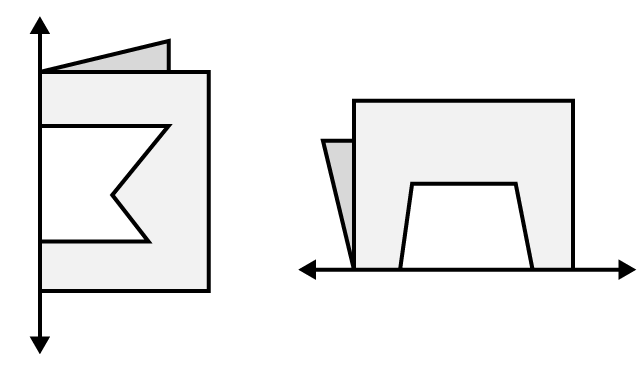Solutions:

We may draw a diagram of the complete figure as follows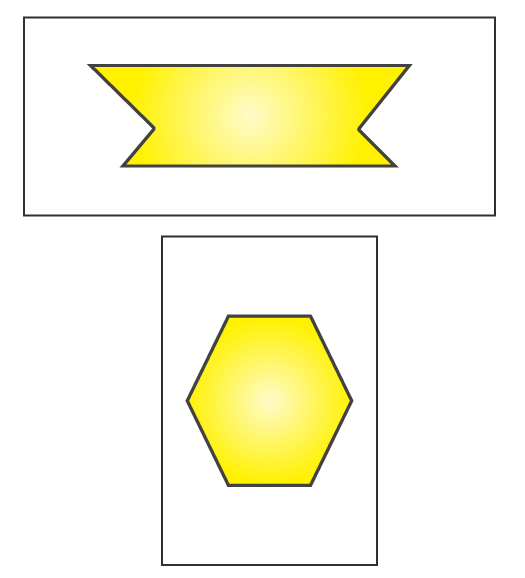## Exercise 13.3

1. Find the number of lines of symmetry in each of the following shapes. How will you check your answers?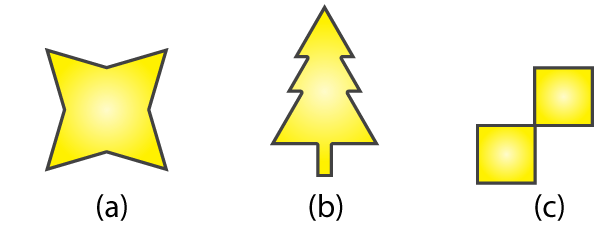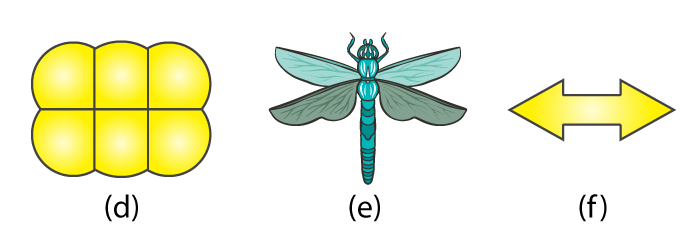Solutions:

(a) For the given figure there are 4 lines of symmetry.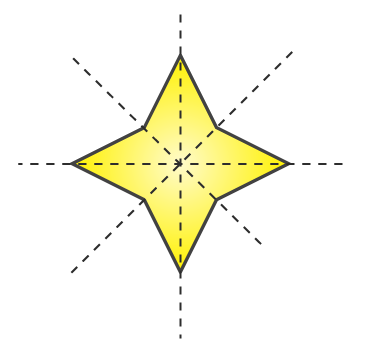(b) For the given figure there is only 1 line of symmetry.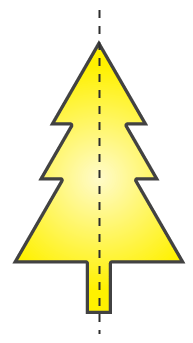(c) For the given figure there are 2 lines of symmetry.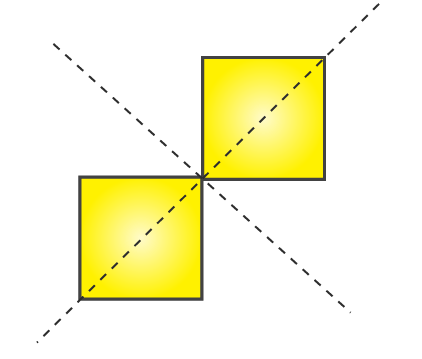(d) For the given figure there are 2 lines of symmetry.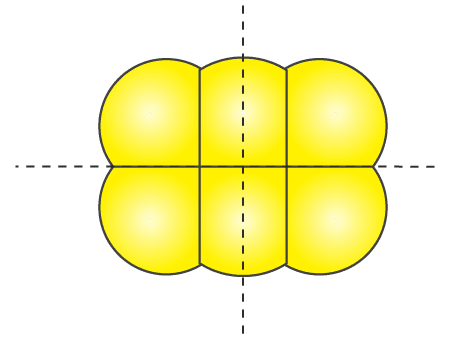(e) For the given figure there is only 1 line of symmetry.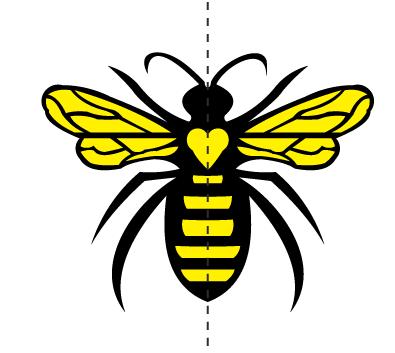(f) For the given figure there are 2 lines of symmetry.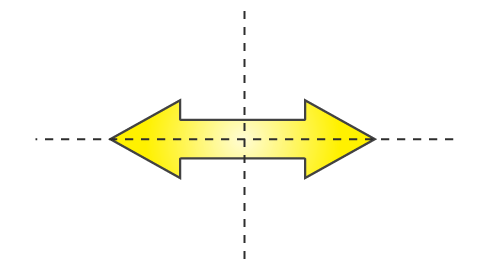2. Copy the following drawing on squared paper. Complete each one of them such that the resulting figure has two dotted lines as two lines of symmetry.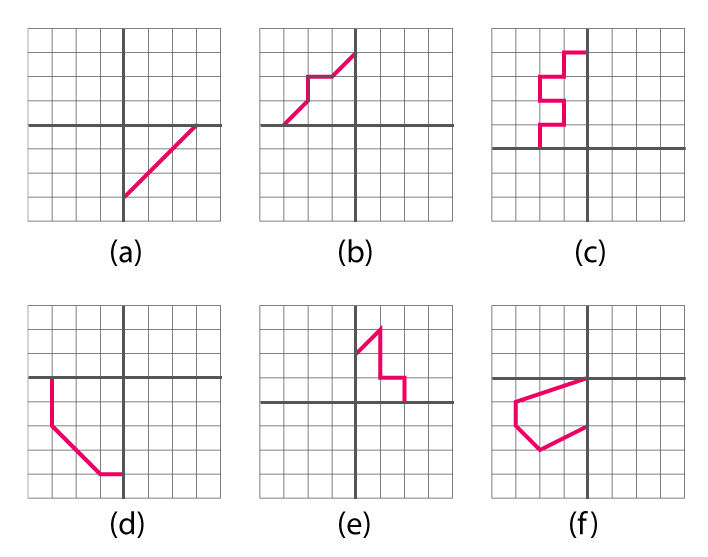How did you go about completing the picture?

Solutions:

We can complete these figures by drawing similar parts as shown in these figures. First about the vertical line of symmetry and then about the horizontal line of symmetry or first about the horizontal line of symmetry and then about the vertical line of symmetry.

Completed figures are as follows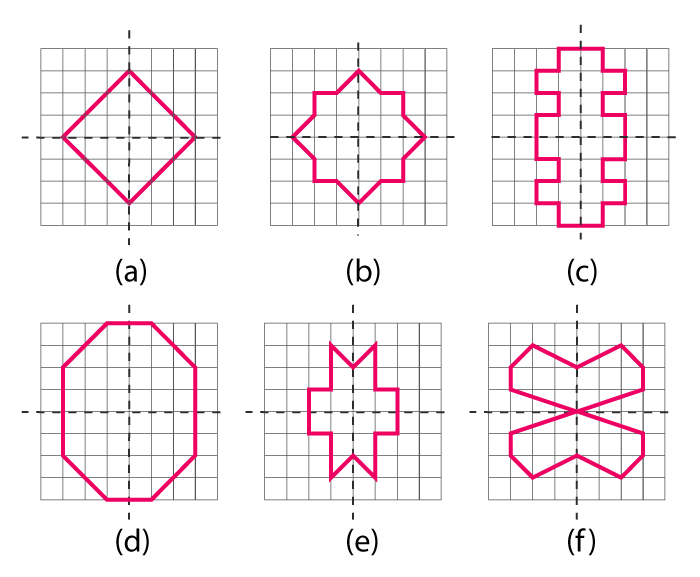3. In each figure alongside, a letter of the alphabet is shown along with a vertical line. Take the mirror image of the letter in the given line. Find which letters look the same after reflection (i.e. which letters look the same in the image) and which do not. Can you guess why?

Try for O E M N P H L T S V X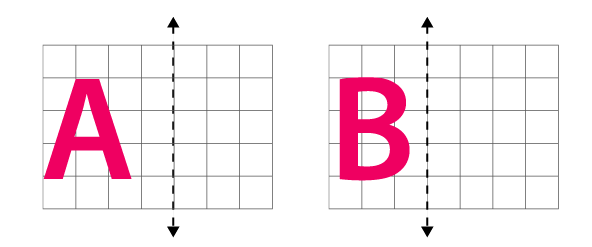Solutions:

The mirror images of these figures are as follows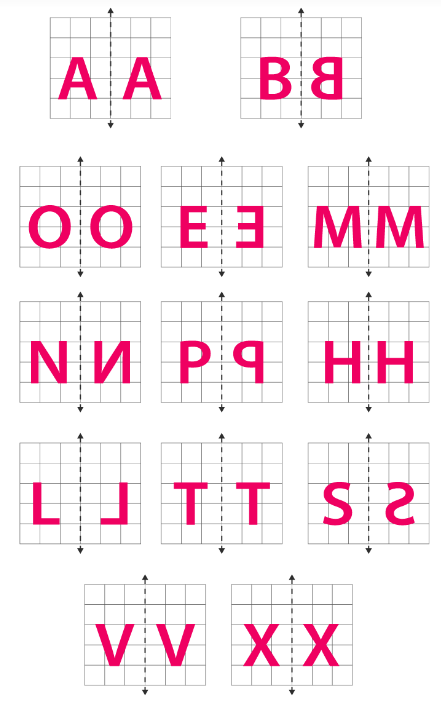The letters having a vertical line of symmetry will have the same mirror images. These letters are O, M, H, T, V, X, and thus these letters will look the same.

I hope you like the information and notes given by us. This will help you a lot in your upcoming exams. Here we are giving ncert solutions to all of you students, through which all of you can score very well in your exams. In this post, we are giving you ncert solutions for class 6 maths. hope you benefit from it. You can download the pdf file of this ncert solutions.

 Whatsapp Group Join
 Telegram channel Join